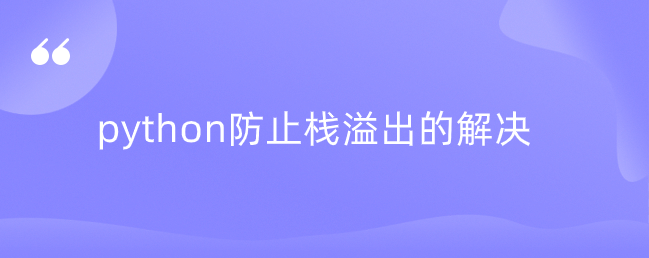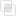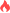python防止栈溢出的解决2021-05-21 16:06:46901浏览 · 0收藏 · 0评论1、说明

2、实例

```def fact(n):
return fact_iter(n, 1)

def fact_iter(num, product):
if num == 1:
return product
return fact_iter(num - 1, num * product)

# fact(5)的调用过程
===> fact_iter(5, 1)
===> fact_iter(4, 5)
===> fact_iter(3, 20)
===> fact_iter(2, 60)
===> fact_iter(1, 120)
===> 120```python集合魔法函数有哪些python实例创建销毁的函数整理python三种属性管理魔法函数python中高斯模糊是什么python如何在二维图像上进行卷积python读取txt文件python如何另起一行输入代码python中mock的断言使用python归并排序的基本思路python快速排序的运作过程python归并排序和快速排序比较python user-agent如何反爬虫python使用time.sleep设置时间间隔python Tkinter模块是什么python爬虫ip池如何实现python美元转换成人民币转换代码python中dict避免key不存在的错误python命名关键字参数的使用注意python如何防止栈溢出python输入身高体重算BMIpython中isprintable判断字符的使用python中有哪些大小写转换方法python中lstrip()截掉字符python partition如何分割字符串splitlines在python中返回列表python copy()和直接赋值的区别python get获取指定键值python in操作符是什么python中popitem如何使用python中update更新字典的方法python异常中else的使用886

python捕获多个异常的规则1181

python ReLU函数是什么1235